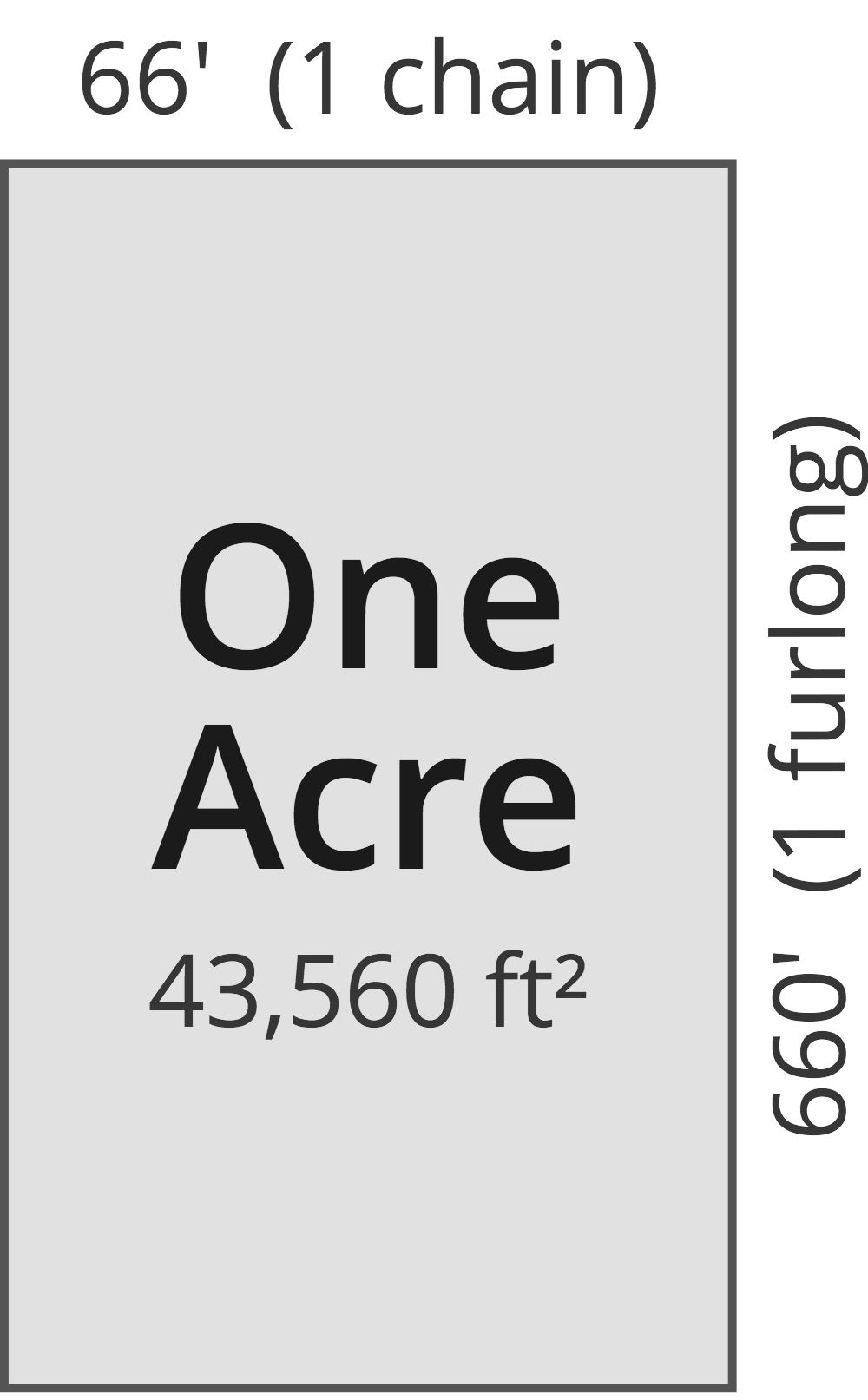# Acres to Square Millimeters Converter

Enter the area in acres below to get the value converted to square millimeters.

(find acres)
Results in Square Millimeters:1 ac = 4,046,856,422.4 sq mm
1 ac = 40,468,564.224 sq cm
1 ac = 4,046.856422 sq m

Do you want to convert square millimeters to acres?

## How to Convert Acres to Square Millimeters

To convert a measurement in acres to a measurement in square millimeters, multiply the area by the following conversion ratio: 4,046,856,422.4 square millimeters/acre.

Since one acre is equal to 4,046,856,422.4 square millimeters, you can use this simple formula to convert:

square millimeters = acres × 4,046,856,422.4

The area in square millimeters is equal to the area in acres multiplied by 4,046,856,422.4.

For example, here's how to convert 5 acres to square millimeters using the formula above.
square millimeters = (5 ac × 4,046,856,422.4) = 20,234,282,112 sq mm

### How Many Square Millimeters Are in an Acre?

There are 4,046,856,422.4 square millimeters in an acre, which is why we use this value in the formula above.

1 ac = 4,046,856,422.4 sq mm

## What is an Acre?

One acre is defined as the area equal to a space that is one chain (66 ft) by one furlong (660 ft), or 10 square chains. That's equal to 43,560 square feet or 1/640 of a square mile for those unfamiliar with those units of measure.The acre is a US customary and imperial unit of area. Acres can be abbreviated as ac; for example, 1 acre can be written as 1 ac.

You can use an acreage calculator to measure the area of a plot of land in acres by locating the boundaries on a map.

## What is a Square Millimeter?

One square millimeter is equivalent to the area of a square with edges that are each 1 millimeter in length. One square millimeter is equal to 0.00155 square inches or 1/100 of a square centimeter.

The square millimeter, or square millimetre, is a multiple of the square meter, which is the SI derived unit for area. In the metric system, "milli" is the prefix for thousandths, or 10-3. A square millimeter is sometimes also referred to as a square mm. Square millimeters can be abbreviated as sq mm, and are also sometimes abbreviated as mm². For example, 1 square millimeter can be written as 1 sq mm or 1 mm².

## Acre to Square Millimeter Conversion Table

Table showing various acre measurements converted to square millimeters.
Acres Square Millimeters
0.000000001 ac 4.0469 sq mm
0.000000002 ac 8.0937 sq mm
0.000000003 ac 12.14 sq mm
0.000000004 ac 16.19 sq mm
0.000000005 ac 20.23 sq mm
0.000000006 ac 24.28 sq mm
0.000000007 ac 28.33 sq mm
0.000000008 ac 32.37 sq mm
0.000000009 ac 36.42 sq mm
0.0000000001 ac 0.404686 sq mm
0.000000001 ac 4.0469 sq mm
0.00000001 ac 40.47 sq mm
0.0000001 ac 404.69 sq mm
0.000001 ac 4,047 sq mm
0.00001 ac 40,469 sq mm
0.0001 ac 404,686 sq mm
0.001 ac 4,046,856 sq mm
0.01 ac 40,468,564 sq mm
0.1 ac 404,685,642 sq mm
1 ac 4,046,856,422 sq mm

## References

1. National Institute of Standards and Technology, Specifications, Tolerances, and Other Technical Requirements for Weighing and Measuring Devices, Handbook 44 - 2019 Edition, https://nvlpubs.nist.gov/nistpubs/hb/2019/NIST.HB.44-2019.pdf